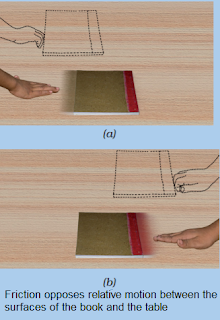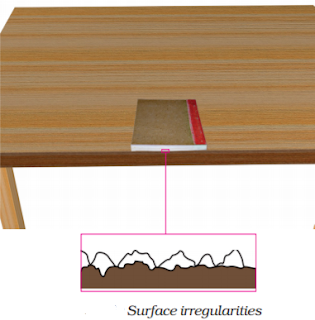#### Study Material and Notes of Ch 12 Friction Class 8th Science

Topics in the chapter

• Introduction
• Friction
→ Causes of Friction
→ Nature of surfaces of Friction
→ Friction when object is pressed hard
→ Friction according to mass of object
→ Examples where friction is useful
→ Examples where friction is harmful
• Rolling Friction
• Sliding Friction
• Fluid Friction

Introduction

→ The vehicles, any object, moving over the surface of another object slows down due to the force of friction applied on it.

Friction→ It is an opposing force that acts between surfaces in contact moving with respect to each other.

→ It always opposes relative motion between two surfaces.

Cause of friction→ Friction is cause by the irregularities on the two surfaces in contact.

→ We are able to walk because of the force of friction.

Nature of surfaces for Friction

Smooth surfaces: less friction
Rough surfaces: greater friction

Friction when object is pressed hard

Greater pressing force means Greater friction.

Friction according to mass of object

Greater mass: Greater friction

Sliding friction < Static friction
Rolling friction < Sliding friction

Examples where friction is useful(i) Walking
(ii) Handling any object
(iii) Rolling motion of ball or wheel

Examples where friction is harmful(i) Energy dissipation of engine due to friction between surfaces in motion.
(ii) More energy is lost in pulling or pushing an object in rough surfaces.
(iii) Wear and tear of shoe soles and tyres.(iv) Lubrication: Powder on carom board, oil in machine
(v) Wheel: Wheels reduce friction (because rolling friction < sliding friction).
(vi) Shoe soles and tires are threaded to increase friction for a better grip.
(vii) Fluid friction is minimised by giving suitable shapes to vehicles moving through fluids.

Rolling Friction→ When a body rolls over the surface of another body, the resistance to its motion is called rolling friction.

Sliding Friction

→ When a body slides over the surface of another body, the resistance to its motion is called sliding friction.

• Rolling friction < Sliding friction

→ Ball bearings change sliding friction into rolling friction.

Fluid Friction

→ Fluid friction is minimised by giving suitable shapes to vehicles moving through fluids.

• Fluid friction is also called drag.

→ Friction force depends upon the speed of the object with respect to the fluid.

→ Friction force depends upon the shape of the and nature of the fluid.